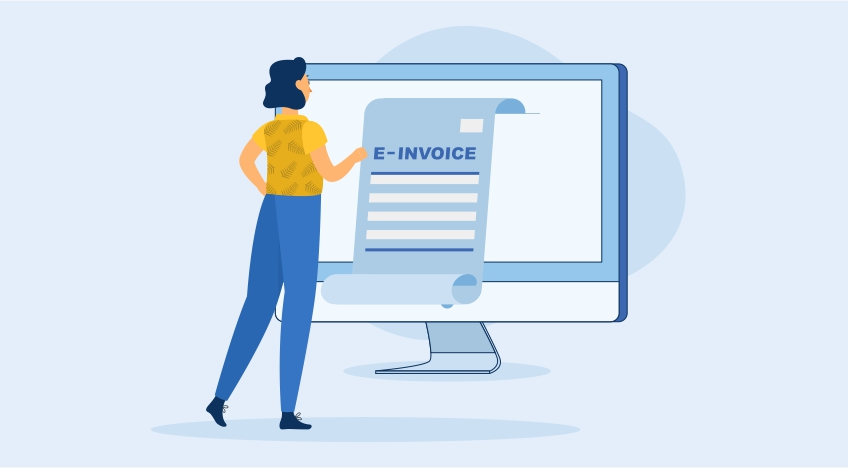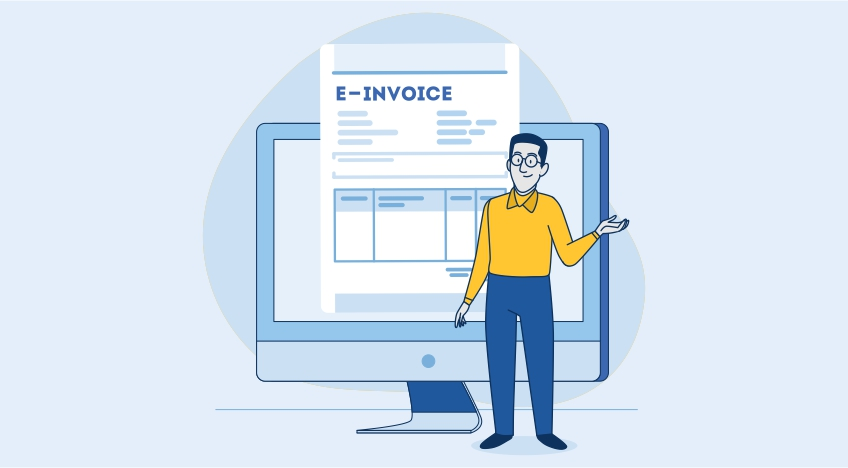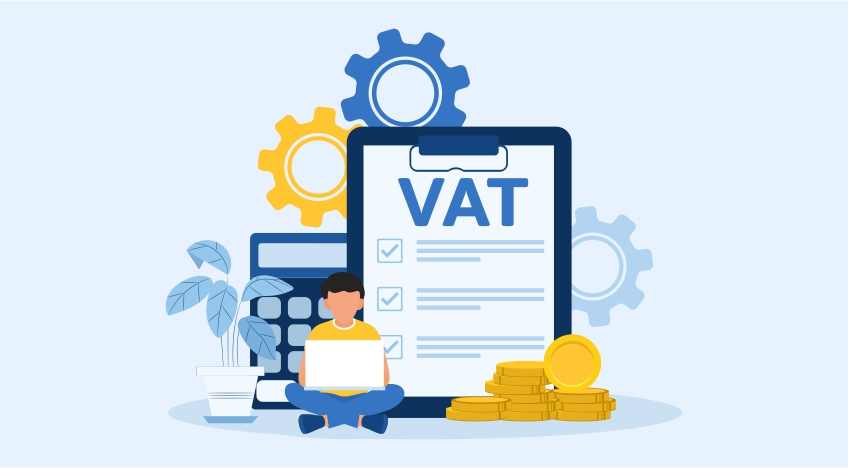# How to Calculate VAT In Uganda | VAT Calculator

## VAT calculation in Uganda

In Uganda, there are two types of calculation methods available to calculate VAT: VAT-inclusive calculators and VAT-exclusive calculators. These use different formulae for calculation. The VAT-inclusive calculator lets you know how much VAT was applied to the product while the VAT-exclusive calculator tells you how much you paid for VAT. You can also calculate VAT yourself using the VAT-inclusive and VAT-exclusive formulas.

## VAT-inclusive calculation

VAT-inclusive calculation denotes that the price of the product is inclusive of VAT. That is, the final price of the product includes VAT so you do not need to pay VAT separately. When you get a bill from the store, the VAT will be separately shown to you on each product. This enables you to understand how much VAT was applied to each product that you purchased. The standard rate of VAT on taxable supplies is 18% and  if you buy zero-rated supplies, then you will see 0% VAT applied.

## Formula of VAT-inclusive calculation

The VAT calculator Uganda mostly uses the following formula where the VAT amount is calculated by multiplying VAT-inclusive price with the VAT rate divided by 100 plus the VAT rate.

VAT amount = (VAT-inclusive price) x (VAT rate / (100 + VAT rate))

Where;

VAT amount is VAT in UGX that was applied to arrive at the final product price.

VAT-inclusive price is the total price that you paid when you purchased the product.

VAT rate is the rate of the product as per the URA and it is generally 18% for standard taxable supplies.

## Examples of VAT-inclusive calculation

Example 1

Let us say you purchased shoes for UGX 200,000 where the VAT of 18% has already been applied. Now we want to find out how much VAT you paid in terms of UGX. By using the VAT-inclusive formula, we will find the VAT amount in UGX.

VAT amount = (VAT-inclusive price) x (VAT rate / (100 + VAT rate))

VAT amount = (200,000) x (18 / (100 + 18))

VAT amount = (200,000) x (18/118)

VAT amount = 30,000

This means the total price of the shoes you purchased was UGX 200,000 and you paid VAT of UGX 30,000 which was included in the final price of the shoes. The original price of the shoes was UGX 170,000.

Example 2

You purchased a laptop bag worth UGX 80,000 and you want to know how much VAT you paid for the product. Let us say that the VAT rate is 18%. Here is how you will go about it. First, using the following formula, we will plug in the values for the VAT-inclusive price and the VAT rate.

VAT amount = (VAT-inclusive price) x (VAT rate / (100 + VAT rate))

VAT amount = (80,000) x (18 / (100 + 18))

VAT amount = (80,000) x (18/ 118)

VAT amount = 12,203

You paid a VAT of UGX 12,203 when you paid UGX 80,000 for the laptop bag. The original price or the taxable value of the laptop bag is UGX 67,797.

## VAT-exclusive calculation

VAT-exclusive means the product price does not have VAT included in it. Therefore, the product price is not the final price because you have to pay VAT additionally. When you purchase VAT-exclusive products, two things will be displayed on the bill - the product price excluding  the VAT and  the VAT applicable to the product .The VAT-exclusive calculation involves adding the VAT amount to the price of the product to arrive at the final price with VAT.

## Formula of VAT-exclusive calculation

When you use the VAT calculator in Uganda you will need to enter the rate of VAT and the VAT-exclusive product price that you paid. There are two components of performing the VAT-exclusive calculation. First, you need to calculate how much VAT will be applied to the product by multiplying the VAT-exclusive product price by the VAT rate divided by 100.

VAT amount = (VAT-exclusive product price) x (VAT rate / 100)

Then to arrive at the final product price that is VAT-inclusive, you will add the VAT amount calculated to the VAT-exclusive product price.

Final product price = VAT amount + VAT-exclusive product price

## Examples of VAT- exclusive calculation

Example 1

Let us say a VAT of 18% has to be applied to a sofa that costs UGX 700,000. You want to find out the total amount that you will need to pay. As you have the VAT rate and the VAT-exclusive product price, this is how you will go about calculating the total price to pay.

VAT amount = (VAT-exclusive product price) x (VAT rate / 100)

VAT amount = (700,000) x (18/100)

VAT amount = 700,000 x 0.18

VAT amount = 126,000

This means the total VAT that you will need to pay for the UGX 700,000 sofa is UGX 126,000. Now we will calculate the final product price.

Final product price = VAT amount + VAT-exclusive product price

Final produce price = 126,000 + 700,000

Final product price = 826,000

This means the total that you need to pay for the sofa in addition to the original VAT-exclusive price is UGX 826,000.

Example 2

Let us assume that you are going to purchase a center table for UGX 350,000 and the VAT rate that will be applied will be 18%. We will calculate how much VAT in UGX you need to pay for this table as follows.

VAT amount = (VAT-exclusive product price) x (VAT rate / 100)

VAT amount = (350,000) x (18/100)

VAT amount = 350,000 x 0.18

VAT amount = 63,000

This means according to the 18% VAT rate; you will need to pay a VAT total of UGX 63,000. Next, we will arrive at the final product price as follows.

Final product price = VAT amount + VAT-exclusive product price

Final produce price = 350,000 + 63,000

Final product price = 4,13,000

The total or the final price of the center table will be UGX 4,13,000 after VAT has been added to it. This is the VAT-inclusive price because now the VAT amount has been added to the pure price of the product.

What you must remember when calculating VAT-inclusive and VAT-exclusive calculations is that you will deal with decimals. In Uganda, the standard rate of VAT for taxable supplies is 18% and so it is likely that decimals will be involved. If you are not using a VAT calculator then you can round it off to three decimal points as done in the examples above. This will make your calculations easier. You must also ensure that you know which supplies are exempt and which are zero-rated supplies because no VAT is applied to such products.

#### Latest BlogsHow to Generate e-Invoice in Uganda?

Tally Solutions   Nov-09-2022How to Generate e-Invoice in Tanzania

Tally Solutions   Nov-04-2022e-Invoice Formats in Kenya

Tally Solutions   Nov-04-2022Value Added Tax in Uganda | VAT in Uganda

Tally Solutions   Oct-12-2022VAT Registration In Uganda

Tally Solutions   Oct-12-2022